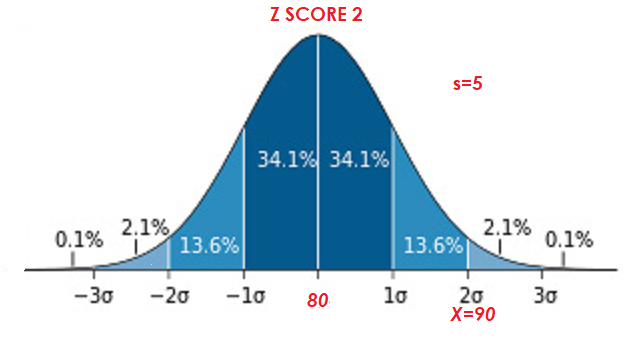Today May 12, 2023.......... 1.1K unique readers ...... 12K requests.......... official Cloudflare stats ..........

# FAQ Frequently asked questions in Statistics

### List of frequently asked question in Statistics

Here is a list of frequently asked question in Statistics by students, researchers, and often by statisticians.

### Q. My experiment has three groups. Can I run t-tests to test the difference between mean1 and mean2, mean1 and mean3, mean2 and mean3?

No, you can not run repeated t-test. The t-distribution takes into account the application of the test only once. Run a one-way ANOVA.

### Q: What is the formula for degrees of freedom df?

The formula for degrees of freedom (df) is: number of scores that goes into the calculation of the mean minus 1. Important note: In ANOVA we calculate the mean of means. Here df=number of means that goes into the calculation of the mean minus 1.
br>

### Q: What is the formula for the standard normal curve?

The formula that creates the standard normal curve is:
$$y={{1\overσ\sqrt{2π}}}e^{-{(χ-μ)^2}\over2σ^2}$$

### Q: What are z scores?

A z score is the standard deviation at which a score lies. The formula for the calculation of the z score is:
$$z={(X-\bar{X})\over{s}}$$ Example: A distribution with mean 80 and standard deviation 5, what is the z sore for score 90? z=90-80/5=10/5=2### Q: What are small-n designs?

Small-n designs are non-statistical experimental designs that employ very few subjects. They focus on detailed observations of the behavior of each subject rather than averages and analysis of subject variability. They were introduced by behaviorists of the Skinnerian persuasion.

### Q: What are post-hoc tests?

Post-hoc tests, also called "a posteriori" tests, are statistical tests used for individual comparisons of means after an analysis of variance (ANOVA) has been performed. They are all based on the logic of the need to make it more difficult for attaining statistical significance, since theoretically the more tests one performs the greater the probability of finding significance.
Here is a list of the most frequently used post--hoc tests:
Duncan's new multiple range test (MRT)
Dunn's Multiple Comparison Test.
Fisher's Least Significant Difference (LSD)
Newman-Keuls.test
Scheffé's test
.........Next
Rate it: